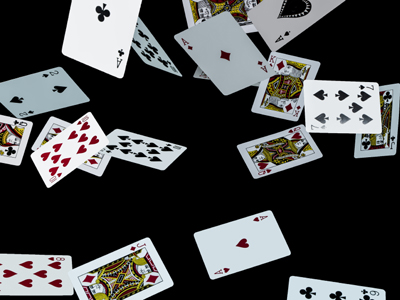Find out about Jessie's house of cards in this quiz!

# Algebra - Percentage Operations (Basic)

This Math quiz is called 'Algebra - Percentage Operations (Basic)' and it has been written by teachers to help you if you are studying the subject at middle school. Playing educational quizzes is a fabulous way to learn if you are in the 6th, 7th or 8th grade - aged 11 to 14.

It costs only \$12.50 per month to play this quiz and over 3,500 others that help you with your school work. You can subscribe on the page at Join Us

You've already learned about percentages and how to convert decimal points into percentages. If you recall, a percentage is simply a way in which you can express a fraction in portions of 100. In this way if you were to see an item for sale at 100% off, it would mean that the item was free. If it was on sale at 90% off, you would multiply the original sales price, let’s say the original price was \$12.00, by .90 as .90 is the same as 90/100 or 90%. Remember that the symbol for percent (%) is converted to a decimal point by moving the decimal point two spaces to the left. Now getting back to the problem, it would read:

\$12.00 x .90 = \$10.80

You now know 90% of \$12.00 is \$10.80. Now you subtract \$10.80 from \$12.00 and you will then learn what the new sale price of the item that you will be purchasing is. So, \$12.00 - \$10.80 = \$1.20. The new sale price is \$1.20.

This is great when you know all of the numbers but what happens when you don’t know all of the numbers? Remember, an unknown number is also referred to as being a variable. When you see a variable being used, you know that the problem you will have to work out will be part of an Algebraic (or Algebra) problem. See below for more detail.

Go straight to Quiz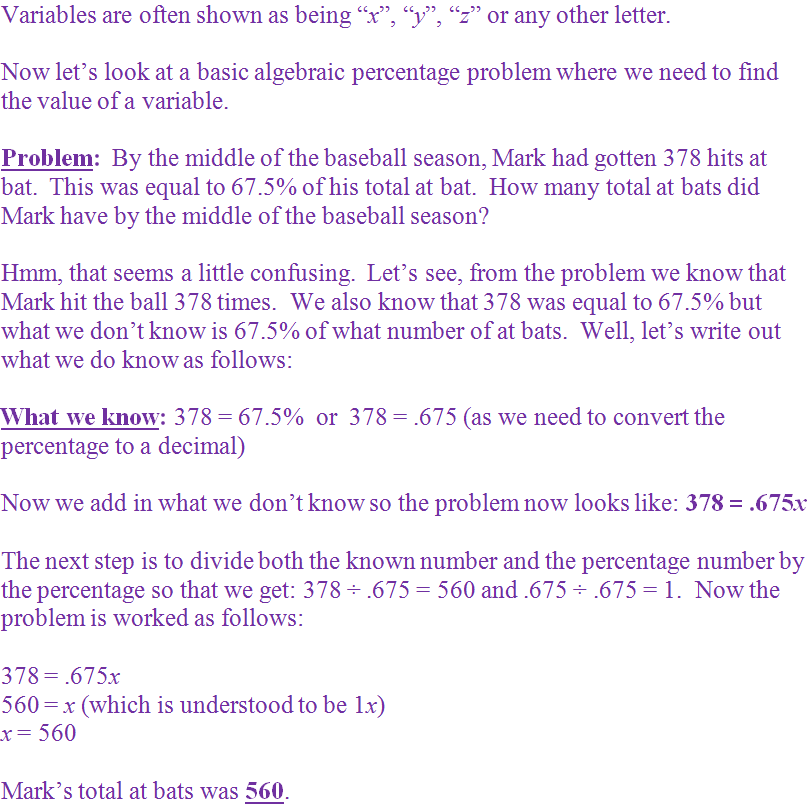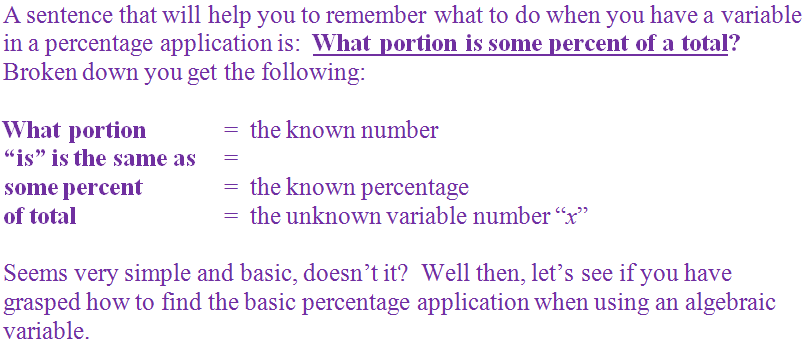1.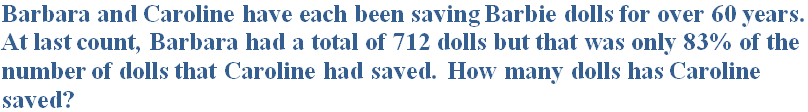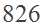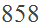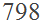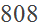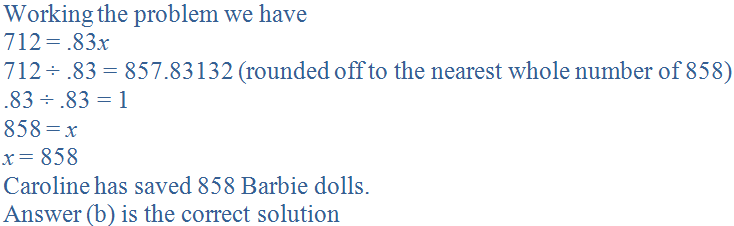2.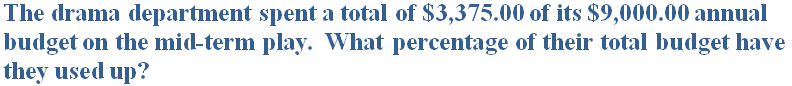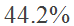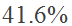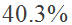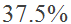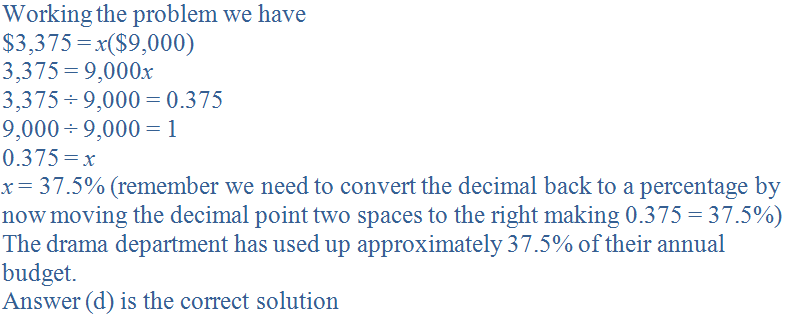3.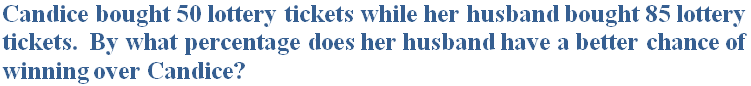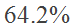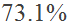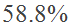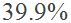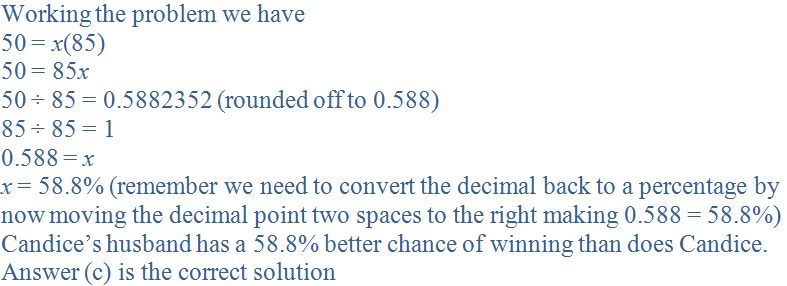4.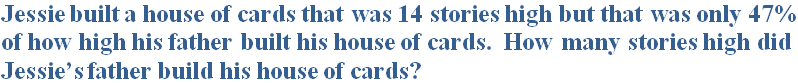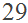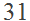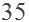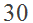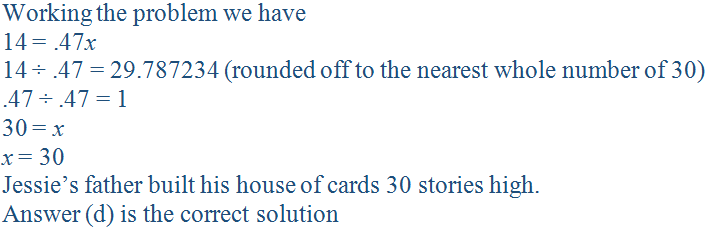5.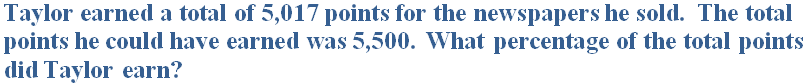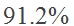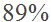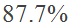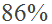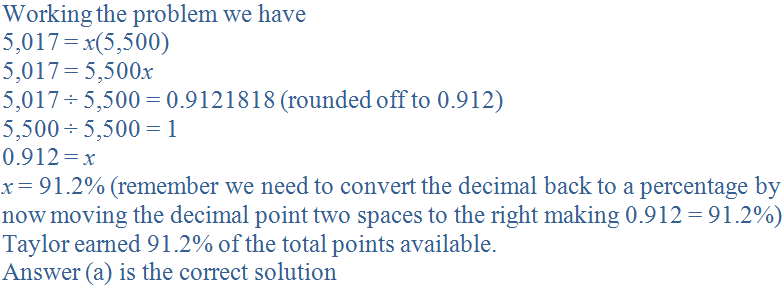6.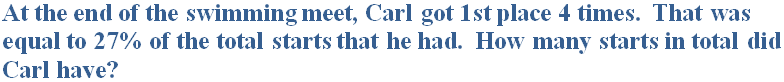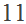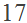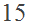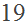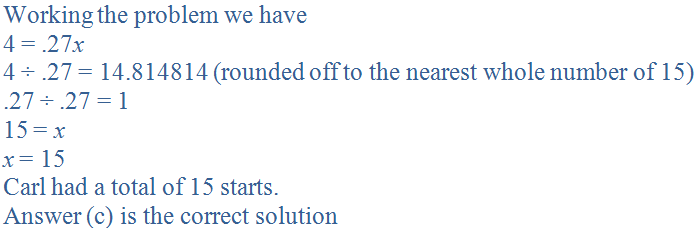7.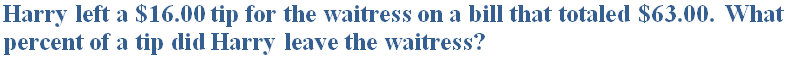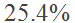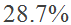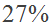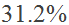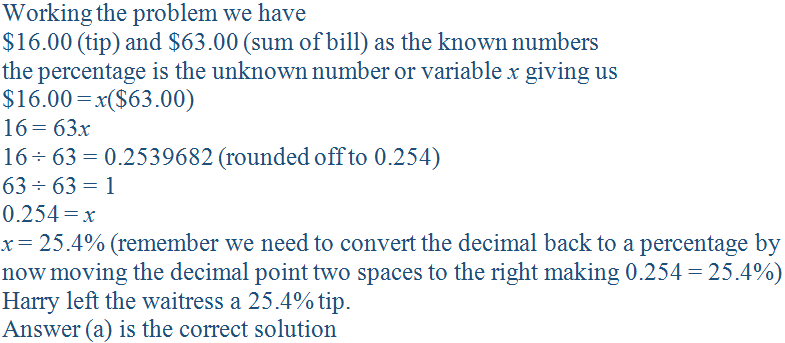8.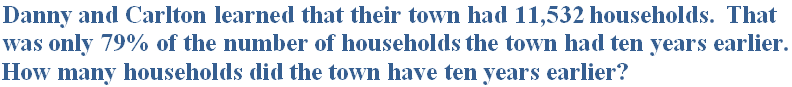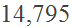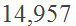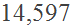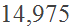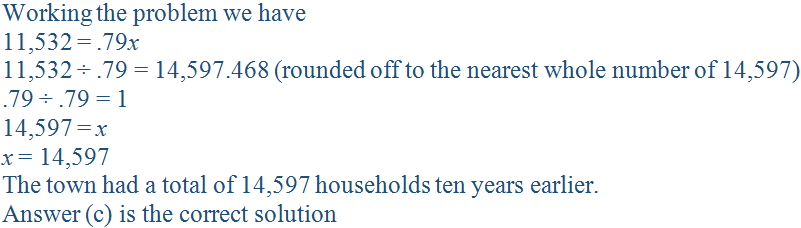9.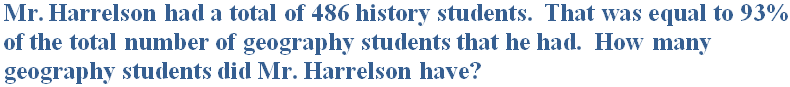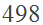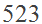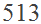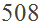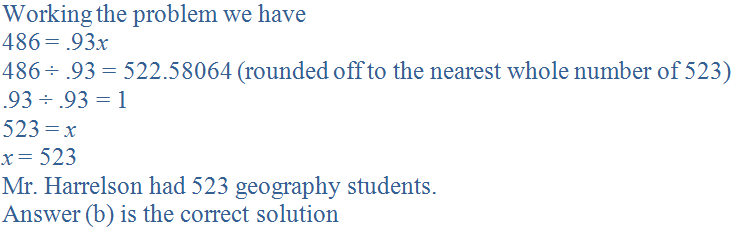10.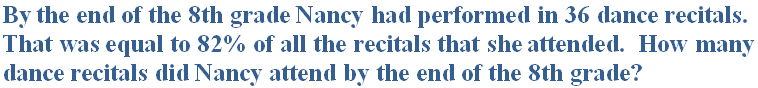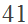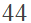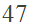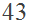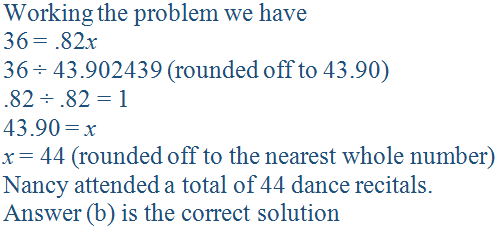Author:  Christine G. Broome